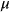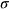# samshareef595

Lv1

## Sam Shareef

0 Followers
0 Following
0 Helped

Published5

### Subjects

Precalculus1Calculus2Mathematics1Statistics1

Assume that females have pulse rates that are normally distributed with a mean of= 72.0 beats per minute and a standard deviation of= 12.5 beats per minute.

Complete parts (a) through (c) below.

a. If 1 adult female Is randomly selected, find the probability that her pulse rate is less than 78 beats per minute.

The probability is ____.

(Round to four decimal places as needed.)

b. If 16 adult females are randomly selected. find the probability that they have pulse rates with a mean less than 78 beats per minute.

The probability is ____.

(Round to four decimal places as needed.)

c. Why can the normal distribution be used in part (b), even though the sample size does not exceed 30?

◯ A. Since the mean pulse rate exceeds 30, the distribution of sample means is a normal distribution for any sample size.

◯ B. Since the original population has a normal distribution, , the distribution of sample means is a normal distribution for any sample size.

◯ C. Since the distribution is of sample means, not individuals, the distribution is a normal distribution for any sample size.

◯ D. Since the distribution is of individuals, not sample means, the distribution is a normal distribution for any sample size.

Answer: a. We are given that the pulse rates of females are normally distribut...
Answer: To find the critical points of the function f(x, y), we need to find t...
Answer: To find the critical points of F(x), we need to find the values of x w...
Answer: The area of the shaded sector is given by the formula:Step-by-step exp...
Answer: To begin, we can rewrite the given equation in standard form:(x - 2)^2...